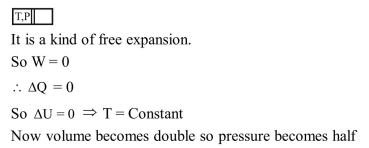# A container with insulating walls is divided into two equal parts by a partition fitted with a valve.

Question:

A container with insulating walls is divided into two equal parts by a partition fitted with a valve. One part is filled with an ideal gas at a pressure $P$ and temperature T, whereas the other part is completely evacuated. If the valve is suddenly opened, the pressure and temperature of the gas will be :-

1. $\frac{\mathrm{P}}{2}, \mathrm{~T}$

2. $\frac{\mathrm{P}}{2}, \frac{\mathrm{T}}{2}$

3. $\mathrm{P}, \mathrm{T}$

4. $\mathrm{P}, \frac{\mathrm{T}}{2}$

Correct Option: 1

Solution: